# OQPSK Demodulator Baseband

Demodulation using OQPSK method

• Library:
• Communications Toolbox / Modulation / Digital Baseband Modulation / PM

•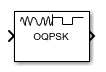## Description

The OQPSK Demodulator Baseband block applies pulse shape filtering to the input waveform and demodulates it using the offset quadrature phase shift keying (OQPSK) method. For more information, see Pulse Shaping Filter. The input is a baseband representation of the modulated signal.

For information about delays incurred by modulator-demodulator pair processing, see Modulation Delays.

## Ports

### Input

expand all

Input baseband waveform, specified as a discrete-time complex scalar or column vector.

The block processes the input signal based on the Output type setting.

Data Types: `double`
Complex Number Support: Yes

### Output

expand all

Output data, returned as an integer or bit column vector.

## Parameters

expand all

### Modulation

Output type, specified as `Integer` or `Bit`.

• When you set Output type to `Integer`, the block outputs a vector of integer symbols with values from 0 to 3, the length of which is the number of output symbols.

• When you set Output type to `Bit`, the block outputs a 2-bit binary representation of integers, in a binary-valued, even-length vector.

The input period for each integer or bit pair is the Samples per symbol times the output sample period.

Phase offset from π/4, specified as a scalar in radians. The phase offset is applied to the zeroth point of the signal constellation before delay of quadrature component. After the OQPSK imaginary-component delay the signal is normalized with unity power.

Example: Setting Phase offset (rad) to `pi/4` aligns the zeroth point of the QPSK signal constellation point on the axes, {(1,0), (0,j), (-1,0), (0,-j)}.

Signal constellation bit mapping, specified as `Gray`, `Binary`, or a custom 4-element numeric vector of integers with values from 0 to 3.

SettingConstellation Mapping for IntegersConstellation Mapping for BitsComment

`Gray`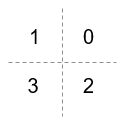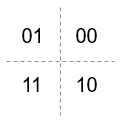The signal constellation mapping is Gray-encoded.

`Binary`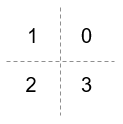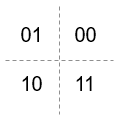The signal constellation mapping for the input integer `m` (0 ≤ `m` ≤ 3) is the complex value ```e(j*(PhaseOffset+π/4) + j*2*π*m/4)```.

Custom 4-element numeric vector of integers with values from 0 to 3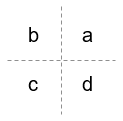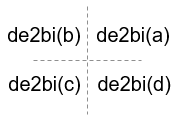Elements [a b c d] must be composed of the set of values [0, 1, 2, 3] in any order.

### Filtering

Select the filtering pulse shape: ```Half sine```, `Normal raised cosine`, `Root raised cosine`, or `Custom`.

Raised cosine filter rolloff factor, specified as a scalar from `0` to `1`.

#### Dependencies

This property is enabled when Pulse shape is ```Normal raised cosine``` or ```Root raised cosine```.

Data Types: `double`

Filter length in symbols, specified as a scalar. An ideal raised cosine filter has an infinite impulse response. However, to realize a practical implementation of this filter, the object truncates the impulse response to Filter span (in symbols) symbols.

#### Dependencies

This property is enabled when Pulse shape is ```Normal raised cosine``` or ```Root raised cosine```.

Data Types: `double`

Filter numerator, specified as a row vector.

#### Dependencies

This parameter is enabled when Pulse shape is `Custom`.

Data Types: `double`

Number of samples per symbol, specified as a positive even integer.

Data Types: `double`

### Other Parameters

• `Enforce single-rate processing` — Executes the model, ensuring that the input and output signals have the same port sample time. The block implements the rate change by making a size change at the output when compared to the input. For integer outputs, the output width equals 1/Samples per symbol times the input width.

• `Allow multirate processing` — Executes the model, allowing the input and output signals to have different port sample times. The output symbol time is Samples per symbol times the input sample time.

Select the output data type: `double`, `single`, or `uint8`.

## Block Characteristics

 Data Types `Boolean` | `double` | `integer` | `single` Multidimensional Signals `no` Variable-Size Signals `no`

expand all

## Extended Capabilities

### Topics

Introduced before R2006a